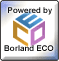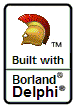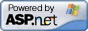Delphi Clinic C++Builder Gate Training & Consultancy Delphi Notes Weblog Dr.Bob's Webshop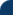These are the voyages using Delphi Enterprise (and Architect). Its mission: to explore strange, new worlds. To design and build new applications. To boldly go...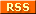Title: Author: Bob Swart Posted: 3/18/2010 1:38:56 PM (GMT+1) Content: Dynamic arrays always start at index 0, but sometimes people ask me if you really cannot have a dynamic array that starts at 1. Sure you can, if you write a little wrapper around it...Here's a simple TDynamicInteger class, using a default array property, with a getter and setter that subtract 1 from the given index (so we can use 1, but it will actually be 0).`type TDynamicInteger = class private FArray: Array of Integer; function GetValue(Index: Integer): Integer; procedure SetValue(Index, Value: Integer); function GetLength: Integer; procedure SetLength(const Value: Integer); public constructor Create(Size: Integer); destructor Destroy; override; public property Length: Integer read GetLength write SetLength; property Value[index: integer]: Integer read GetValue write SetValue; default; end; (* TDynamicInteger *) constructor TDynamicInteger.Create(Size: Integer); begin inherited Create; System.SetLength(FArray, Size) end; destructor TDynamicInteger.Destroy; begin FArray := nil; inherited; end; function TDynamicInteger.GetLength: Integer; begin Result := System.Length(FArray) end; procedure TDynamicInteger.SetLength(const Value: Integer); begin System.SetLength(FArray, Value) end; function TDynamicInteger.GetValue(Index: Integer): Integer; begin Result := FArray[Index-1] // 1-based array end; procedure TDynamicInteger.SetValue(Index, Value: Integer); begin FArray[Index-1] := Value // 1-based array end; `Note that we can set the length using the argument of the constructor, or just by assigning a value to the Length property. Example usage: ` var X: TDynamicInteger; initialization X := TDynamicInteger.Create(4); // X.Length := 4; X := 1; X := 2; X := 3; X := 4; finalization X.Free end. `In my upcoming Delphi 2010 Development Essentials I will use this example and even turn it into a template class so you can have 1-based arrays of any type! Back